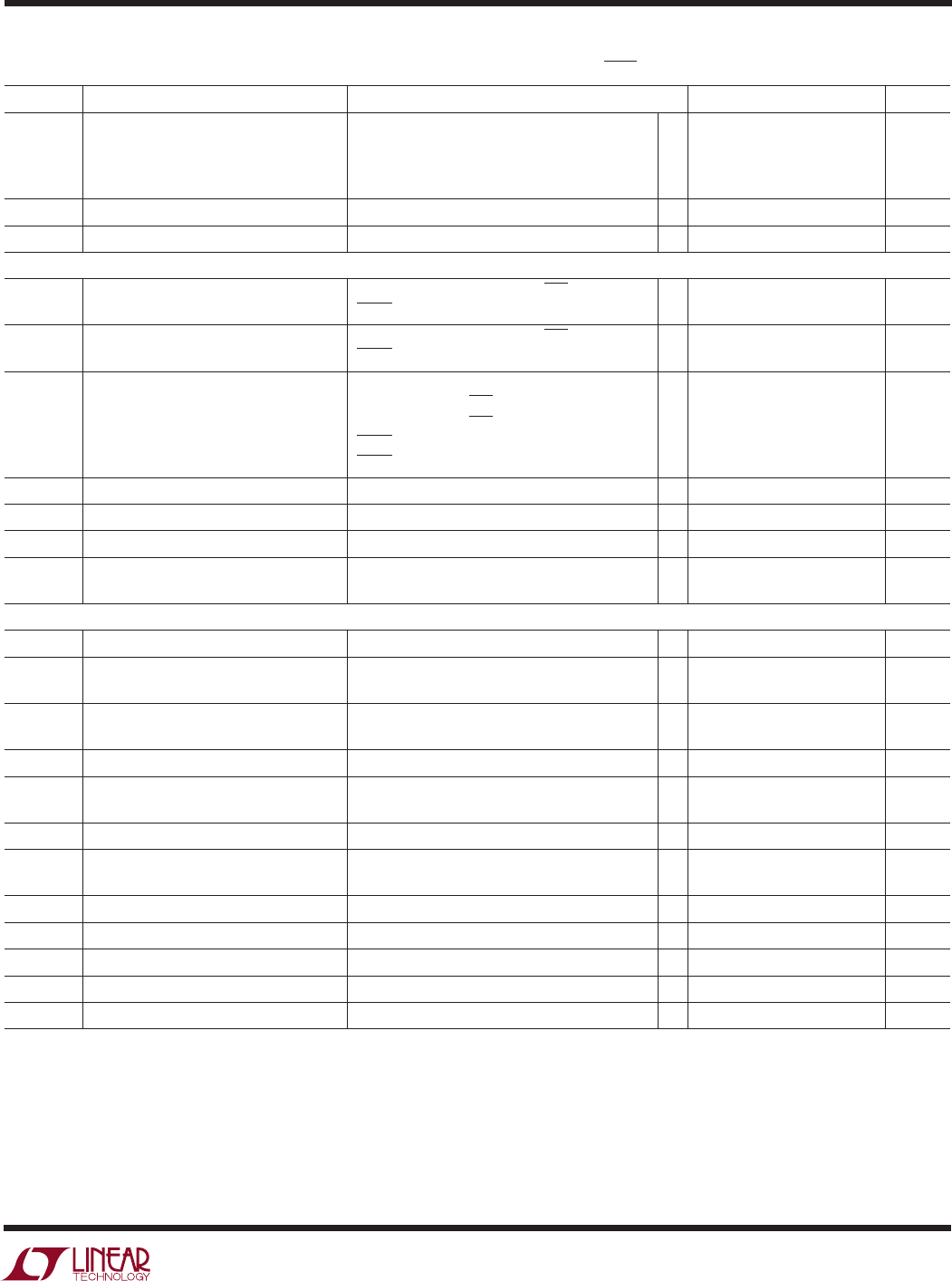# Datasheet3
LTC2846
sn2846 2846fs
The denotes specifications which apply over the full operating
temperature range, otherwise specifications are at T
A
= 25°C. V
CC
= 5V, V
IN
= 3.3V, V
SHDN
= V
IN
, unless otherwise noted. (Notes 2, 3)
ELECTRICAL CHARACTERISTICS
SYMBOL PARAMETER CONDITIONS MIN TYP MAX UNITS
V
Negative Charge Pump Output Voltage V.28 Mode, No Load 9.6 V
7.5 8.5 V
V.35 Mode
5.5 6.5 V
RS530, RS530-A, X.21 Modes, Full Load
4.5 6.0 V
f
OSC
Charge Pump Oscillator Frequency 500 kHz
t
r
Charge Pump Rise Time No-Cable Mode/Power-Off to Normal Operation 2 ms
Logic Inputs and Outputs
V
IH
Logic Input High Voltage D1, D2, D3, M0, M1, M2, DCE/DTE 2.0 V
SHDN 2.4 V
V
IL
Logic Input Low Voltage D1, D2, D3, M0, M1, M2, DCE/DTE 0.8 V
SHDN 0.5 V
I
IN
Logic Input Current D1, D2, D3 ±10 µA
M0, M1, M2, DCE/DTE = GND
30 75 120 µA
M0, M1, M2, DCE/DTE = V
IN
±10 µA
SHDN = GND ±0.1 µA
SHDN = 3V 16 32 µA
V
OH
Output High Voltage I
O
= –3mA 2.7 3 V
V
OL
Output Low Voltage I
O
= 1.6mA 0.2 0.4 V
I
OSR
Output Short-Circuit Current 0V V
O
V
IN
±50 mA
I
OZR
Three-State Output Current M0 = M1 = M2 = V
IN
, V
O
= GND –30 –85 –160 µA
M0 = M1 = M2 = V
IN
, V
O
= V
IN
±10 µA
V.11 Driver
V
ODO
Open Circuit Differential Output Voltage R
L
= 1.95k (Figure 1) ± 5V
V
ODL
L
= 50 (Figure 1) 0.5V
ODO
0.67V
ODO
V
R
L
= 50 (Figure 1) ±2V
V
OD
Change in Magnitude of Differential R
L
= 50 (Figure 1) 0.2 V
Output Voltage
V
OC
Common Mode Output Voltage R
L
= 50 (Figure 1) 3V
V
OC
Change in Magnitude of Common Mode R
L
= 50 (Figure 1) 0.2 V
Output Voltage
I
SS
Short-Circuit Current V
OUT
= GND ±150 mA
I
OZ
Output Leakage Current V
A
and V
B
0.25V, Power Off or ±1 ±100 µA
No-Cable Mode or Driver Disabled
t
r
, t
f
Rise or Fall Time (Figures 2, 13) 21525 ns
t
PLH
Input to Output Rising (Figures 2, 13) 15 40 65 ns
t
PHL
Input to Output Falling (Figures 2, 13) 15 40 65 ns
t Input to Output Difference, t
PLH
– t
PHL
(Figures 2, 13) 0312 ns
t
SKEW
Output to Output Skew (Figures 2, 13) 3 ns